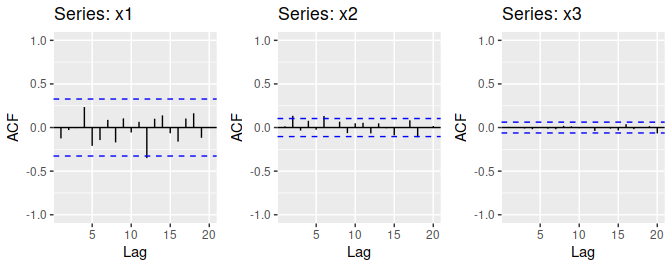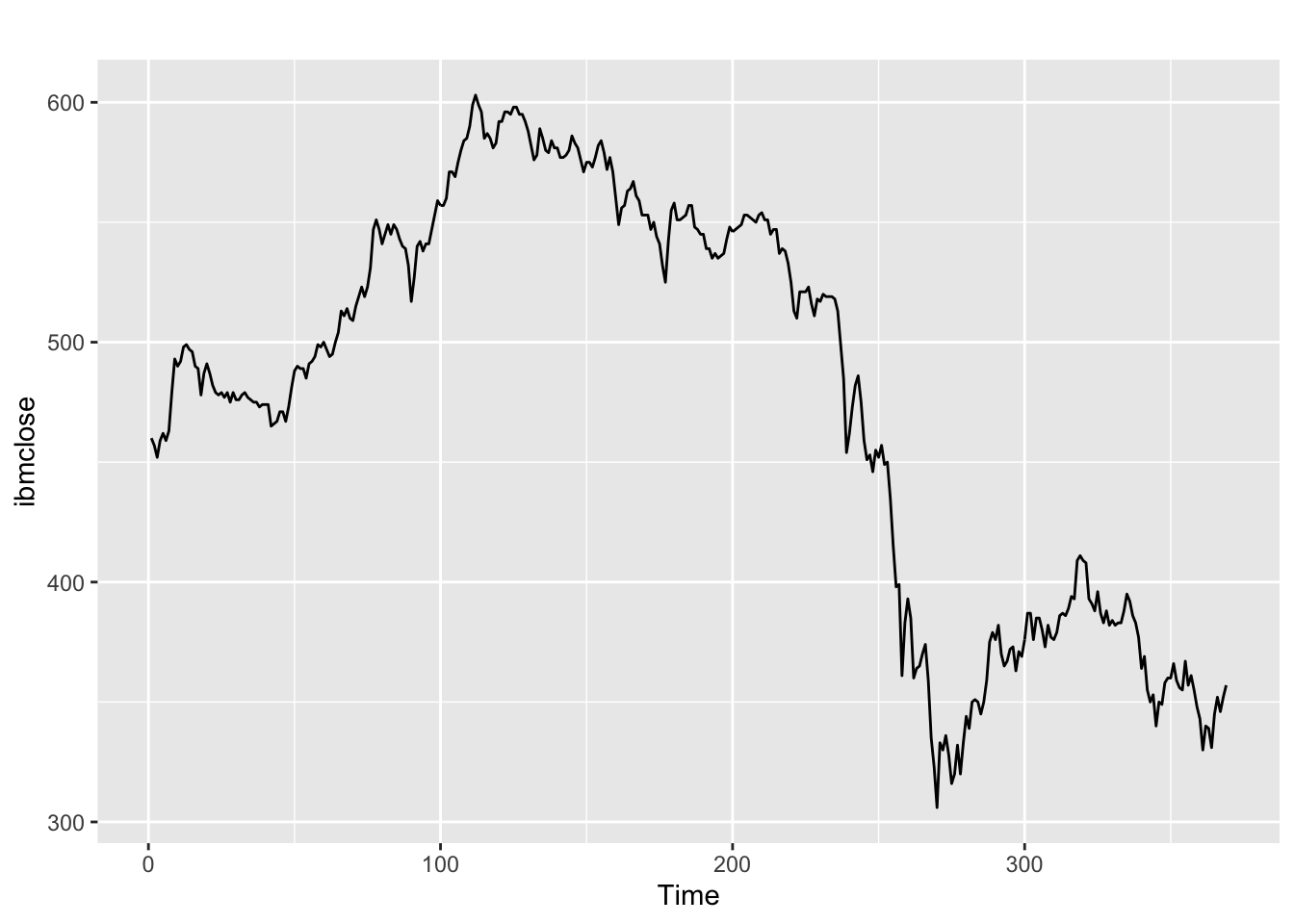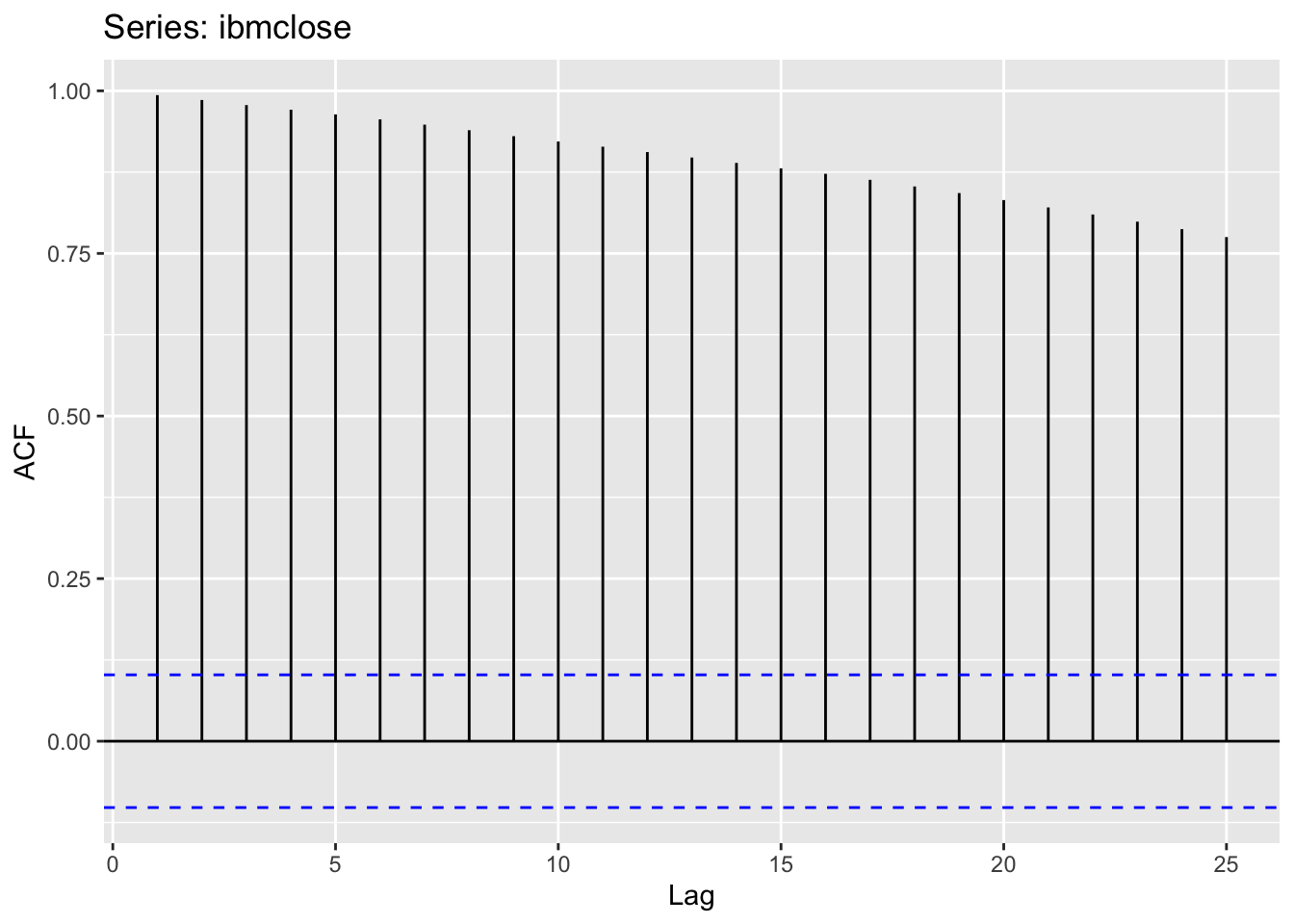## Problem 8.1

Figure 8.31 shows the ACFs for 36 random numbers, 360 random numbers and 1,000 random numbers.

1. Explain the differences among these figures. Do they all indicate that the data are white noise?Problem 8.1 ACF Graphs

ACF looks for correlations between values in a sequence. It’s intended to be used with timeseries data where there is an assumption that some value is changing over time. To calculate a given lag value for \(N\), the algorithm goes back thru all the list of values and picks out every \(Nth\) value and does a correlation analysis. With fewer values in the list, we see a stronger correlation (random chance). As the number of vlaues in the list increase, when calculating the correlation, we are reverting to a mean where there should be 0 correlation (since we are drawing random numbers). With only 36 values, we are more likely to see spurious correleations, but as we tend to 1000 values, spurious correlations smooth out and are less likely to be noted as possibly significant.

The more historic data we provide, the more likely we will find real lag correlations. The less histoic data we provide, the greater the chance we find correlations we cannot trust.

For smaller lags, \(N\), we have more values (e.g. \(N=2\) and 36 numbers, we have 18 lag values) and are less likely to see a significant correlation. As \(N\) increases, (e.e. \(N=15\) and 36 numbers, we have 2 lag values), the chance for spurios correlations increases - true for both white noise and real timeseries data.

1. Why are the critical values at different distances from the mean of zero? Why are the autocorrelations different in each figure when they each refer to white noise?

With fewer random numbers in our “timeseries”, each calculated lag value has fewer datapoints when calculating the correlation and the greater the chance that lag value appears correlated. With greater numbers of lag values in the correlation formula, the correlation revert to mean of 0. Each figure has different autocorrelation merely due to chance and how the random numbers happened to align.

## Problem 8.2

A classic example of a non-stationary series is the daily closing IBM stock price series (data set `ibmclose`). Use R to plot the daily closing prices for IBM stock and the ACF and PACF. Explain how each plot shows that the series is non-stationary and should be differenced.

``autoplot(ibmclose)````ggAcf(ibmclose)````ggPacf(ibmclose)``